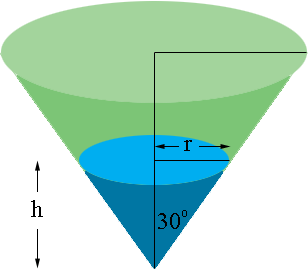SEARCH HOMEMath Central Quandaries & QueriesHi my name is patience and I'm having a problem with this question. Water pours into a conical tank of semi vertical angle 30 degrees at the rate of 4 cm^3/s, where h is the depth of the water at time t. At what rate is the water rising in the tank when h = 10 cm? Thank youHi Patience,

Did you draw a diagram?The volume of water in the tank is $V = \large \frac13 \pi \; r^2 h.$ $V$ and $h$ are both functions of time $t$ and the rate of change of the volume is $\large \frac{dV}{dt}.$

From the diagram what trig function relates $h, r$ and the angle measure of 30 degrees? Use this to express $r$ as a function of $h.$ Substitute into the expression for the volume so that you have $V$ as a function of $h$ alone. Differentiate implicitly with respect to $t$ to obtain a relationship involving $\large \frac{dV}{dt}, \frac{dh}{dt}$ and $h.$ Substitute for $\large \frac{dV}{dt}$ and $h$ and solve for $\large \frac{dh}{dt}.$

PennyMath Central is supported by the University of Regina and The Pacific Institute for the Mathematical Sciences.